### Home > MC1 > Chapter 6 > Lesson 6.2.2 > Problem6-61

6-61.

Copy and complete each of the Diamond Problems below. The pattern used in the Diamond Problems is shown at right.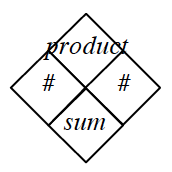1.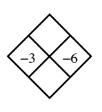This problem is the same as the other diamond problems, but the $\#$ boxes are now labeled ''$x$'' and ''$y$''.

Add and then multiply $−3$ and $−6$ to fill the empty boxes.

1.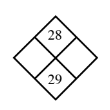What numbers add to $29$ and multiply to $28$?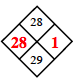See the diamond above.

1.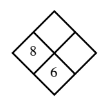Begin by subtracting $8$ from $6$ to find ''$y$''. Then multiply $8$ by ''$y$'' to find the product.